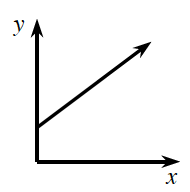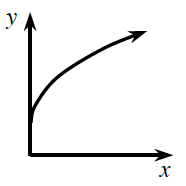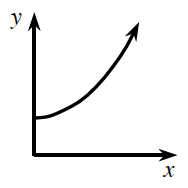Home > APCALC > Chapter 1 > Lesson 1.2.4 > Problem1-76

1-76.

Each of the continuous functions in the table below is increasing, but each increases differently. Match each graph below with the function that grows in a similar fashion in the table.

 $x$ $1$ $2$ $3$ $4$ $5$ $6$ $7$ $8$ $9$ $f (x)$ $15.5$ $19.0$ $22.5$ $26.0$ $29.5$ $33.0$ $36.5$ $40.0$ $43.5$ $g(x)$ $1$ $2$ $4$ $8$ $16$ $32$ $64$ $128$ $256$ $h(x)$ $12$ $76$ $108$ $124$ $132$ $136$ $138$ $139$ $139.5$
1.2.3.Use the eTool below to create the graphs of the functions.
Click the link at right for the full version of the eTool: Calc 1-76 HW eTool